NEET  >  NCERT Exemplar: Nuclei - 1

# NCERT Exemplar: Nuclei - 1 - Notes | Study Physics Class 12 - NEET

 1 Crore+ students have signed up on EduRev. Have you?

MULTIPLE CHOICE TYPE QUESTIONS I

Q.1. Suppose we consider a large number of containers each containing initially 10000 atoms of a radioactive material with a half life of 1 year. After 1 year,
(a) All the containers will have 5000 atoms of the material.
(b) All the containers will contain the same number of atoms of the material but that number will only be approximately 5000.
(c) The containers will in general  have different numbers of the atoms of the material but their average will be close to 5000.
(d) None of the containers can have more than 5000 atoms.
Ans. (c)
Solution.
Half life time for a radioactive substance is defined as the time in which a radioactive atomic substance remains half of its original value of radioactive atom. So after one year means one half life i.e., average atoms of radioactive substance remain after 1 Year in each container is equal to 1/2 of 10,000 = 5000 atoms (average).

Q.2. The gravitational force between a H-atom and another particle of mass m will be given by Newton’s law: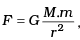where r is in km and
(a) M = mproton + melectron
(b)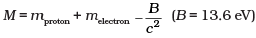(c) M is not related to the mass of the hydrogen atom.
(d)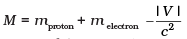(|V| = magnitude of the potential energy of electron in the H-atom).
Ans.
(b)
Solution.
During formation of H-atom some mass of nucleons convert into energy by E = mc2, this energy is used to bind the nucleons along with nucleus. So mass of atom becomes slightly less than sum of actual masses of nucleons and electrons.
Actual mass of H atom = MP + Me - (B.E./c2) (B/c2 is binding energy)
B.E. (B) of H atoms is 13.6 eV per atom.

Q.3. When a nucleus in an atom undergoes a radioactive  decay, the electronic energy levels of the atom
(a) Do not change for any type of radioactivity
(c) Change for α-radioactivity but not for others
(d) Change for β-radioactivity but not for others
Ans.
(b)
Solution.
β- Particles carries one unit of negative charge, and α-particle carries 2 units of positive charge, and Υ-particle carries no charge. So the electronic energy level of the atom changes in emission of α and β particle, but not in Υ decay.

Q.4. Mx and My denote the atomic masses of the parent and the daughter nuclei respectively in a radioactive decay. The Q-value for a β decay is Q1 and that for a β+ decay is Q2. If me denotes the mass of an electron, then which of the following statements is correct?
(a) Q1 = (Mx – My) c2 and Q2 = (Mx – My – 2me)c2
(b) Q1 = (Mx – My) c2 and Q2 = (Mx – My )c2
(c) Q1 = (Mx – My – 2me) c2 and Q2 = (Mx – My +2 me)c2
(d) Q1 = (Mx – My + 2me) c2 and Q2 = (Mx – My +2 me)c2
Ans.
(a)
Solution.
Key concept: Q value or energy of nuclear reaction: The energy absorbed or released during a nuclear reaction is known as Q-value of nuclear reaction.
Q-value = (Mass of reactants – mass of products)c2 Joules
= (Mass of reactants – mass of products) amu
If Q < 0, the nuclear reaction is known as endothermic. (The energy is absorbed in the reaction)
If Q > 0, the nuclear reaction is known as exothermic. (The energy is released in the reaction)
Let the nucleus be ZX4.
β- decay is represented as: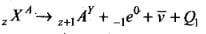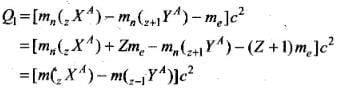⇒ Q1 = (M- My)c2
β+ decay is represented as: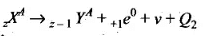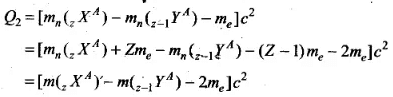⇒ Q2 = (M- M-2me)c2

Q.5. Tritium is an isotope of hydrogen whose nucleus Triton contains 2 neutrons and 1 proton. Free neutrons decay into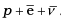. If one of the neutrons in Triton decays, it would transform into He3 nucleus. This does not happen. This is because
(a) Triton energy is less than that of a He3 nucleus.
(b) The electron created in the beta decay process cannot remain in the nucleus.
(c) Both the neutrons in triton have to decay simultaneously resulting in a nucleus with 3 protons, which is not a He3 nucleus.
(d) Because free neutrons decay due to external perturbations which is absent in a triton nucleus
Ans.
(a)
Solution.
Triton (1H3) has 1 proton and 2 neutrons. If a neutron decays as: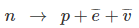, then nucleus will have 2 proton and 1 neutron, i.e. triton atom converts in 2He3 (2 proton and 1 neutron).
Binding energy of 1H3 is much smaller than 2He3  so transformation is not possible energetically.

Q.6. Heavy stable nuclei have more neutrons than protons. This is because of the fact that
(a) Neutrons are heavier than protons.
(b) Electrostatic force between protons are repulsive.
(c) Neutrons decay into protons through beta decay.
(d) Nuclear forces between neutrons are weaker than that between protons
Ans.
(b)
Solution.
Key concept: Neutron-proton ratio (N/2 ratio:) The chemical properties of atom are governed entirely by the number of protons (Z ) in the nucleus, stability of an atom appears to depend on both the number o f protons and number of neutrons.
(i) For lighter nuclei, the greatest stability is achieved when the number o f protons and neutrons are approximately equal (N = Z ), i.e. N/Z = 1
(ii) Heavy nuclei are stable only when they have more neutrons than protons. Thus heavy nuclei are neutron rich compared to lighter nuclei ( for heavy nuclei, more is the number of protons in the nucleus, greater is the electrical repulsive force between them. Therefore more neutrons are added to provide the strong attractive forces necessary to keep the nucleus stable.)

Q.7. In a nuclear reactor, moderators slow down the neutrons which come out in a fission process. The moderator used have light nuclei. Heavy nuclei will not serve the purpose because
(a) They will break up.
(b) Elastic collision of neutrons with heavy nuclei will not slow them down.
(c) The net weight of the reactor would be unbearably high.
(d) Substances with heavy nuclei do not occur in liquid or gaseous state at room temperature
Ans.
(b)
Solution.
Key concept: A moderator is a material used in a nuclear reactor to slow down the neutrons produced from fission. By slowing the neutrons down the probability of a neutron interacting with Uranium-235 nuclei is greatly increased thereby maintaining the chain reaction. Moderators are made from materials with light nuclei which do not absorb the neutrons but rather slow them down by a series of collisions.
The moderator only slows neutrons down in order to increase the interaction with Uranium nuclei. They do not give any protection if the reaction goes out of control. 1 fa chain reaction is heading out of control the reactors needs to be able to reduce the concentration of neutrons. For this the reactor uses control rods. Control rods are matte from material with the ability to absorb neutrons. Cadmium and Boron are examples of suitable materials. By inserting.control rods between the fuel rods the chain reaction can be slowed dowp-or shut down. Withdrawing the control rods can restart or speed up the reaction.
In our given question, the moderator used have light nuclei (like proton). When protons undergo perfectly elastic collision with the neutron emitted their velocities are exchanged, i.e., neutrons come to rest and protons move with the velocity of neutrons.
Heavy nuclei will not serve the purpose because elastic collisions of neutrons with heavy nuclei will not slow them down.

MULTIPLE CHOICE TYPE QUESTIONS II

Q.8. Fusion processes, like combining two deuterons to form a He nucleus are impossible at ordinary temperatures and pressure.
The reasons for this can be traced to the fact:
(a) Nuclear forces have short range
(b) Nuclei are positively charged
(c) The original nuclei must be completely ionized before fusion can take place
(d) The original nuclei must first break up before combining with each other
Ans.
(a, b)
Solution.
Two deuteron can combine to form He atom when their nuclei come close to nuclear range where electrostatic repulsive force between positively charged deuterons does not act. Electrostatic force increases very high on decreasing their distance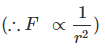To overcome this electrostatic repulsive force nuclei need very high temperature and pressure. Hence to combine two nuclei, they must reach closer of the range of where nuclear force acts and electrostatic repulsive force does not act verifies the answer (a) and (b).

Q.9. Samples of two radioactive nuclides A and B are taken. λA and λB are the disintegration constants of A and B respectively. In which of the following cases, the two samples can simultaneously have the same decay rate at any time?
(a) Initial rate of decay of A is twice the initial rate of decay of B and λA = λB
(b) Initial rate of decay of A is twice the initial rate of decay of B and λA > λB
(c) Initial rate of decay of B is twice the initial rate of decay of A and λA > λB
(d) Initial rate of decay of B is same as the rate of decay of A at t = 2h and λB < λA
Ans.
(b, d)
Solution.
Key concept:
Law of radioactive disintegration : According to Rutherford and Soddy law for radioactive decay is as follows:
“At any instant the rate of decay of radioactive atoms is proportional to the number of atoms present at that instant.” i.e.
dN/dt ∞ N ⇒ dN/dt = -λN
it can be proved that N=N0e-λ1
In terms of mass M— M0e-λ1
where N = Number of atoms remains undecayed after time t,
N0 = Number of atoms present initially (i.e., at t = 0),
M = Mass of radioactive nuclei at time t,
M0 = Mass of radioactive nuclei at time t = 0,
N0-N= Number of disintegrated nucleus in time t,
dN/dt= rate of decay, λ = Decay constant or disintegration constant or radioactivity constant or Rutherford Soddy’s constant or the probability of decay per unit time of a nucleus.
The samples of the two radioactive nuclides A and B can simultaneously have the same decay rate at any time if initial rate of decay of A is twice the initial rate of decay of B and λA > λB.
Also, when initial rate of decay of B is the same as rate of decay of A at t = 2h and λB < λA.

Q.10. The variation of decay rate of two radioactive samples A and B with time is shown in Figure.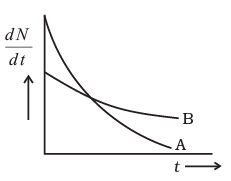Which of the following statements are true?
(a) Decay constant of A is greater than that of B, hence A always decays faster than B.
(b) Decay constant of B is greater than that of A but its decay rate is always smaller than that of A.
(c) Decay constant of A is greater than that of B but it does not always decay faster than B.
(d) Decay constant of B is smaller than that of A but still its decay rate becomes equal to that of A at a later instant.

Ans. (c, d)
Solution.
From the given graph slope of A is greater than B so rate of decay of A is greater than of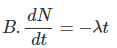or instant t or for a particular time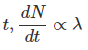so λ> λB.  at point P the intersecting point of two graphs at time t same.

Q.11. He23 and He13 nuclei have the same mass number. Do they have the same binding energy?
Ans.
The nuclei He23 and He13 have the same mass number. He23 has two protons and one neutron. He23 has one proton and two neutrons. As He3 has only one proton hence the repulsive force between protons is missing in 1He3, so the binding energy of 1He3 is greater than that of 2He3.

Q.12. Draw a graph showing the variation of decay rate with number of active nuclei.
Ans.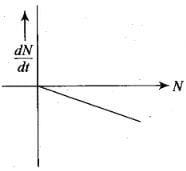According to Rutherford and Soddy law for radioactive decay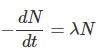where decay constant (λ) is constant for a given radioactive material. Therefore, graph between N and dN/dt is a straight line as shown in the diagram.

Q.13. Which sample, A or B shown in Figure has shorter mean-life?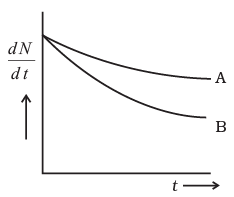Ans.
Initially at t=0 from figure given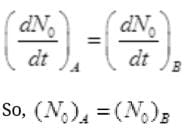i.e., initially both samples has equal number of radioactive atoms. Considering at any instant t = t from figure,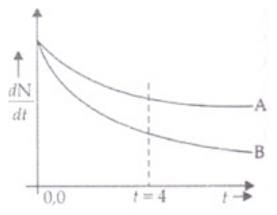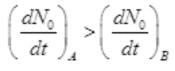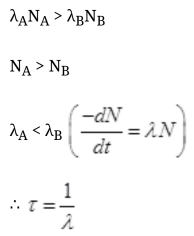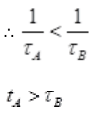So mean life time of sample A is greater than of B.

Q.14. Which one of the following cannot emit radiation and why?  Excited nucleus, excited electron.
Ans.
Excited electron cannot emit radiation because energy of electronic energy levels is in the range of eV and not MeV (mega electron volt), y-radiations have energy of the order of MeV.
Key concept: The energy of internal motion of a nucleus is quantized. A typical nucleus has a set of allowed energy levels, including a ground state (state of lowest energy) and several excited states. Because of the great strength of nuclear interactions, excitation energies of nuclei are typically of the order of the order of 1 MeV, compared with a few eV for atomic energy levels. In ordinary physical and chemical transformations the nucleus always remains in its ground state. When a nucleus is placed in an excited state, either by bombardment with high-energy particles or by a radioactive transformation, it can decay to the ground state by emission of one or more photons called gamma rays or gamma-ray photons, with typical energies of 10 keV to 5 MeV. This process is called gamma (γ) decay.

Q.15. In pair annihilation, an electron and a positron destroy each other to produce gamma radiation. How is the momentum conserved?
Ans.
when an electron and positron combine together coming from opposite directions they destroy each other by the emission of two Υ-rays in opposite direction to conserve linear momentum as below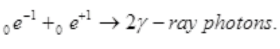The document NCERT Exemplar: Nuclei - 1 - Notes | Study Physics Class 12 - NEET is a part of the NEET Course Physics Class 12.
All you need of NEET at this link: NEET

## Physics Class 12

157 videos|452 docs|213 tests
 Use Code STAYHOME200 and get INR 200 additional OFF

## Physics Class 12

157 videos|452 docs|213 tests

### How to Prepare for NEET

Read our guide to prepare for NEET which is created by Toppers & the best Teachers

Track your progress, build streaks, highlight & save important lessons and more!

,

,

,

,

,

,

,

,

,

,

,

,

,

,

,

,

,

,

,

,

,

;